# US20110007402A1 - Projection variable focus lens and projection display device - Google Patents

## Info

Publication number
US20110007402A1
US20110007402A1 US12/824,973 US82497310A US2011007402A1 US 20110007402 A1 US20110007402 A1 US 20110007402A1 US 82497310 A US82497310 A US 82497310A US 2011007402 A1 US2011007402 A1 US 2011007402A1
Authority
US
United States
Prior art keywords
lens
variable focus
projection
projection variable
movement
Prior art date
Legal status (The legal status is an assumption and is not a legal conclusion. Google has not performed a legal analysis and makes no representation as to the accuracy of the status listed.)
Abandoned
Application number
US12/824,973
Inventor
Masaru Amano
Current Assignee (The listed assignees may be inaccurate. Google has not performed a legal analysis and makes no representation or warranty as to the accuracy of the list.)
Fujinon Corp
Original Assignee
Fujinon Corp
Priority date (The priority date is an assumption and is not a legal conclusion. Google has not performed a legal analysis and makes no representation as to the accuracy of the date listed.)
Filing date
Publication date
Priority to JP2009162501A priority Critical patent/JP5307655B2/en
Priority to JPP2009-162501 priority
Application filed by Fujinon Corp filed Critical Fujinon Corp
Assigned to FUJINON CORPORATION reassignment FUJINON CORPORATION ASSIGNMENT OF ASSIGNORS INTEREST (SEE DOCUMENT FOR DETAILS). Assignors: AMANO, MASARU
Publication of US20110007402A1 publication Critical patent/US20110007402A1/en
Abandoned legal-status Critical Current

• 230000003287 optical Effects 0.000 claims abstract description 61
• 230000014509 gene expression Effects 0.000 claims description 70
• 230000000051 modifying Effects 0.000 claims description 9
• 238000005286 illumination Methods 0.000 claims description 6
• 230000004075 alteration Effects 0.000 abstract description 113
• 201000009310 astigmatism Diseases 0.000 abstract description 29
• 238000010586 diagram Methods 0.000 description 46
• 230000000875 corresponding Effects 0.000 description 35
• 230000005499 meniscus Effects 0.000 description 21
• 239000004973 liquid crystal related substance Substances 0.000 description 7
• 238000004519 manufacturing process Methods 0.000 description 5
• 239000000203 mixture Substances 0.000 description 5
• 230000021615 conjugation Effects 0.000 description 4
• 230000000694 effects Effects 0.000 description 4
• 239000011521 glass Substances 0.000 description 4
• 239000002131 composite material Substances 0.000 description 3
• 239000003086 colorant Substances 0.000 description 1
• 239000011347 resin Substances 0.000 description 1
• 229920005989 resin Polymers 0.000 description 1
• 238000000926 separation method Methods 0.000 description 1

## Images

•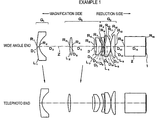•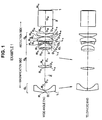•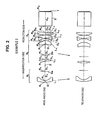•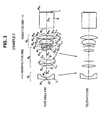•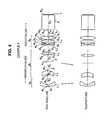•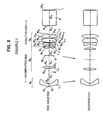•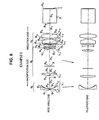•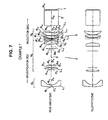•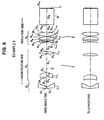•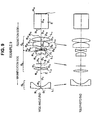•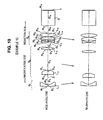•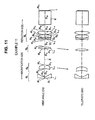•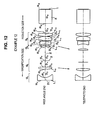•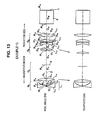•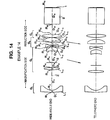•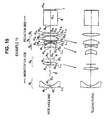•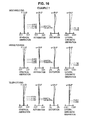•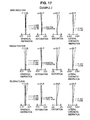••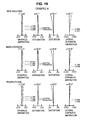•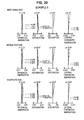•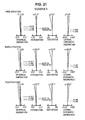••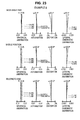•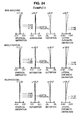•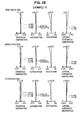•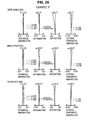•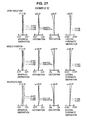•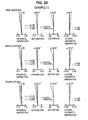••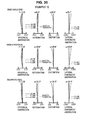•## Classifications

• GPHYSICS
• G02OPTICS
• G02BOPTICAL ELEMENTS, SYSTEMS, OR APPARATUS
• G02B15/00Optical objectives with means for varying the magnification
• G02B15/14Optical objectives with means for varying the magnification by axial movement of one or more lenses or groups of lenses relative to the image plane for continuously varying the equivalent focal length of the objective
• G02B15/16Optical objectives with means for varying the magnification by axial movement of one or more lenses or groups of lenses relative to the image plane for continuously varying the equivalent focal length of the objective with interdependent non-linearly related movements between one lens or lens group, and another lens or lens group
• G02B15/177Optical objectives with means for varying the magnification by axial movement of one or more lenses or groups of lenses relative to the image plane for continuously varying the equivalent focal length of the objective with interdependent non-linearly related movements between one lens or lens group, and another lens or lens group having a negative front lens or group of lenses
• GPHYSICS
• G02OPTICS
• G02BOPTICAL ELEMENTS, SYSTEMS, OR APPARATUS
• G02B15/00Optical objectives with means for varying the magnification
• G02B15/14Optical objectives with means for varying the magnification by axial movement of one or more lenses or groups of lenses relative to the image plane for continuously varying the equivalent focal length of the objective
• G02B15/143Optical objectives with means for varying the magnification by axial movement of one or more lenses or groups of lenses relative to the image plane for continuously varying the equivalent focal length of the objective having three groups only
• G02B15/1435Optical objectives with means for varying the magnification by axial movement of one or more lenses or groups of lenses relative to the image plane for continuously varying the equivalent focal length of the objective having three groups only the first group being negative
• GPHYSICS
• G02OPTICS
• G02BOPTICAL ELEMENTS, SYSTEMS, OR APPARATUS
• G02B15/00Optical objectives with means for varying the magnification
• G02B15/14Optical objectives with means for varying the magnification by axial movement of one or more lenses or groups of lenses relative to the image plane for continuously varying the equivalent focal length of the objective
• G02B15/144Optical objectives with means for varying the magnification by axial movement of one or more lenses or groups of lenses relative to the image plane for continuously varying the equivalent focal length of the objective having four groups only
• G02B15/1445Optical objectives with means for varying the magnification by axial movement of one or more lenses or groups of lenses relative to the image plane for continuously varying the equivalent focal length of the objective having four groups only the first group being negative
• GPHYSICS
• G02OPTICS
• G02BOPTICAL ELEMENTS, SYSTEMS, OR APPARATUS
• G02B15/00Optical objectives with means for varying the magnification
• G02B15/14Optical objectives with means for varying the magnification by axial movement of one or more lenses or groups of lenses relative to the image plane for continuously varying the equivalent focal length of the objective
• G02B15/145Optical objectives with means for varying the magnification by axial movement of one or more lenses or groups of lenses relative to the image plane for continuously varying the equivalent focal length of the objective having five groups only
• G02B15/1455Optical objectives with means for varying the magnification by axial movement of one or more lenses or groups of lenses relative to the image plane for continuously varying the equivalent focal length of the objective having five groups only the first group being negative

## Abstract

An inexpensive projection variable focus lens includes six lenses and three or less lens groups which are moved when power varies, is telecentric, and is capable of effectively reducing all aberrations including astigmatism. Also, a projection display device includes the projection variable focus lens.
The six lenses include a first lens provided closest to a magnification side and having a negative refractive power, and a second lens from the magnification side that has a positive refractive power. The reduction side of a lens system is telecentric. The six lenses are classified into three or more lens groups. Three or less of the three or more lens groups are moved to change a focal length. When the focal length varies from a wide angle end to a telephoto end, at least the second lens is moved from the reduction side to the magnification side along an optical axis.

## Description

CROSS-REFERENCE TO RELATED APPLICATIONS
• This application is based upon and claims the benefit of priority from the Japanese Patent Application No. 2009-162501 filed on Jul. 9, 2009; the entire contents of which are incorporated herein by reference.
• BACKGROUND OF THE INVENTION
• 1. Field of the Invention
• The present invention relates to a variable focus lens that includes six lenses and is provided in, for example, a projection display device, and a projection display device including the variable focus lens, and more particularly, to a small projection variable focus lens and a projection display device which enlarge and project light having image information from a light valve of, for example, a transmissive or reflective liquid crystal display device or a DMD (digital micro-mirror device) display device onto a screen.
• 2. Description of the Related Art
• In recent years, projection display devices using light valves, such as liquid crystal display devices or DMD display devices, have come into widespread use. In particular, a projection display device has been widely used which uses three light valves corresponding to illumination light components of three primary colors, such as R, G, and B, to modulate the illumination light components, and combines the light components modulated by the three light valves using, for example, a color composition prism, and displays an image on a screen through a projection lens.
• In many cases, the projection display device uses as a projection lens a variable focus lens (zoom lens) capable of changing the size of the image projected onto the screen. A telecentric variable focus lens including four lens groups or five lens groups is generally used as the projection variable focus lens. When high performance or a high zoom ratio is required, a variable focus lens including six lens groups is used.
• In general, the variable focus lens includes a large number of lenses in order to achieve high aberration characteristics, ensure telecentricity, and prevent a reduction in contrast or the occurrence of color unevenness. However, when the number of lenses increases, manufacturing costs also increase. Therefore, it is necessary to construct a variable focus lens capable of achieving the objects with a minimum number of lenses.
• In order to meet the requirements, Japanese Patent Nos. 4206769 (corresponding to US 2004/0257644 A) and 4206708 and JP-A-2007-206331 disclose projection variable focus lenses each including six lenses.
• However, in the projection zoom lens including six lenses disclosed in Japanese Patent No. 4206769, four lens groups are moved when power varies. Therefore, a moving mechanism including, for example, a cam becomes complicated, and the weight of the projection zoom lens or the difficulty of manufacture increases, which results in an increase in manufacturing costs.
• In the projection zoom lens including six lenses disclosed in Japanese Patent No. 4206708 and JP-A-2007-206331, when power varies, the variation in astigmatism is too large. Therefore, it is necessary to reduce the variation in astigmatism.
• SUMMARY OF THE INVENTION
• One embodiment of the invention may provide an inexpensive projection variable focus lens that includes six lenses and three or less lens groups moved when power varies, has telecentricity, and is capable of effectively reducing all aberrations including astigmatism, and a projection display device including the projection variable focus lens.
• According to an aspect of the invention, a projection variable focus lens includes six lenses. The six lenses include a first lens and a second lens. The first lens is provided closest to a magnification side and has a negative refractive power. The second lens from the magnification side has a positive refractive power and is telecentric on a reduction side. The six lenses are classified into three or more lens groups. Three or less of the three or more lens groups are moved to change a focal length. When the focal length varies from a wide angle end to a telephoto end, the second lens is moved from the reduction side to the magnification side along an optical axis.
• The three or more lens group may include a first lens group including the first lens. The first lens group may satisfy the following Conditional expression 1:

• −2.5<f 1 /f w<−0.5  [Conditional expression 1]
• (where fw indicates the focal length of the entire system at the wide angle end, and f1 indicates a focal length of the first lens).
• The three or more lens groups may include a second lens group that is arranged on the reduction side of the first lens group. The second lens group may satisfy the following Conditional expression 2:

• 1.0<f 2 /f w<4.0  [Conditional expression 2]
• (where fw indicates the focal length of the entire system at the wide angle end, and f2 indicates a focal length of the second lens).
• The first lens may have at least one aspheric surface.
• The six lenses may include a third lens from the magnification side that has a positive refractive power and includes a convex surface facing the reduction side.
• The six lenses may include a fourth lens, a fifth lens and a sixth lens. The fourth lens from the magnification side has a negative refractive power. The fifth lens from the magnification side has a positive refractive power. The sixth lens from the magnification side has a positive refractive power.
• According to another aspect of the invention, a projection display device includes a light source, a light valve, an illumination optical unit, and the projection variable focus lens according to the above-mentioned aspect. The illumination optical unit guides light emitted from the light source to the light valve. The light valve modulates the light emitted from the light source. The modulated light is projected onto a screen by the projection variable focus lens.
• The meaning of the ‘variable focus lens’ includes a varifocal lens and a zoom lens. The varifocal lens performs a focusing operation to adjust defocusing when the conjugation length is changed due to power variation, unlike the zoom lens.
• The ‘magnification side’ means an object side (screen side). In the case of reduced projection, for convenience, the screen side is also referred to as the magnification side. The ‘reduction side’ means an original image display area side (light valve side). In the case of reduced projection, for convenience, the light valve side is also referred to as the reduction side.
• In the projection variable focus lens according to the above-mentioned aspect of the invention, the first lens arranged closest to the magnification side is a negative lens and the lens group with a negative refractive power is disposed at the head. Therefore, it is possible to ensure a wide angle of view and a large back focal length with a relatively simple structure.
• When power varies, the positive second lens is moved from the reduction side to the magnification side along the optical axis. In this way, the second lens serves a power varying group as well as a correction group, and it is possible to prevent variation in aberration (particularly, astigmatism or field curvature) over the entire power variable range. In addition, it is possible to form a high-performance projection variable focus lens with a small number of lenses.
• That is, since the first lens is a negative lens, both an on-axis light beam and an off-axis light beam are incident on the second lens at a large height. When the second lens is a positive lens, it is possible to largely correct aberration of the off-axis light beam, such as astigmatism. However, in the case of a variable focus lens, the large aberration correction acts as a defect and a large variation in aberration (particularly, astigmatism) occurs due to the movement of the lenses when power varies. However, in the projection variable focus lens according to the above-mentioned aspect of the invention, in order to prevent the variation in aberration when power varies, the second lens is moved from the reduction side to the magnification side when power varies from the wide angle end to the telephoto end. In addition, when power varies, the height of the off-axis light beam incident on the second lens is maintained to be constant. Therefore, it is possible to consistently obtain the effect of correcting off-axis aberration, such as astigmatism. At the same time, it is possible to reduce the amount of astigmatism.
• The projection display device uses the projection variable focus lens according to one aspect of the invention. Therefore, the projection display device has low manufacturing costs and a light weight and can effectively correct all aberrations including astigmatism.
• BRIEF DESCRIPTION OF THE DRAWINGS
• FIG. 1 is a diagram illustrating the structure of a projection variable focus lens according to Example 1 of the invention;
• FIG. 2 is a diagram illustrating the structure of a projection variable focus lens according to Example 2 of the invention;
• FIG. 3 is a diagram illustrating the structure of a projection variable focus lens according to Example 3 of the invention;
• FIG. 4 is a diagram illustrating the structure of a projection variable focus lens according to Example 4 of the invention;
• FIG. 5 is a diagram illustrating the structure of a projection variable focus lens according to Example 5 of the invention;
• FIG. 6 is a diagram illustrating the structure of a projection variable focus lens according to Example 6 of the invention;
• FIG. 7 is a diagram illustrating the structure of a projection variable focus lens according to Example 7 of the invention;
• FIG. 8 is a diagram illustrating the structure of a projection variable focus lens according to Example 8 of the invention;
• FIG. 9 is a diagram illustrating the structure of a projection variable focus lens according to Example 9 of the invention;
• FIG. 10 is a diagram illustrating the structure of a projection variable focus lens according to Example 10 of the invention;
• FIG. 11 is a diagram illustrating the structure of a projection variable focus lens according to Example 11 of the invention;
• FIG. 12 is a diagram illustrating the structure of a projection variable focus lens according to Example 12 of the invention;
• FIG. 13 is a diagram illustrating the structure of a projection variable focus lens according to Example 13 of the invention;
• FIG. 14 is a diagram illustrating the structure of a projection variable focus lens according to Example 14 of the invention;
• FIG. 15 is a diagram illustrating the structure of a projection variable focus lens according to Example 15 of the invention;
• FIG. 16 is a diagram illustrating all aberrations of the projection variable focus lens according to Example 1;
• FIG. 17 is a diagram illustrating all aberrations of the projection variable focus lens according to Example 2;
• FIG. 18 is a diagram illustrating all aberrations of the projection variable focus lens according to Example 3;
• FIG. 19 is a diagram illustrating all aberrations of the projection variable focus lens according to Example 4;
• FIG. 20 is a diagram illustrating all aberrations of the projection variable focus lens according to Example 5;
• FIG. 21 is a diagram illustrating all aberrations of the projection variable focus lens according to Example 6;
• FIG. 22 is a diagram illustrating all aberrations of the projection variable focus lens according to Example 7;
• FIG. 23 is a diagram illustrating all aberrations of the projection variable focus lens according to Example 8;
• FIG. 24 is a diagram illustrating all aberrations of the projection variable focus lens according to Example 9;
• FIG. 25 is a diagram illustrating all aberrations of the projection variable focus lens according to Example 10;
• FIG. 26 is a diagram illustrating all aberrations of the projection variable focus lens according to Example 11;
• FIG. 27 is a diagram illustrating all aberrations of the projection variable focus lens according to Example 12;
• FIG. 28 is a diagram illustrating all aberrations of the projection variable focus lens according to Example 13;
• FIG. 29 is a diagram illustrating all aberrations of the projection variable focus lens according to Example 14;
• FIG. 30 is a diagram illustrating all aberrations of the projection variable focus lens according to Example 15; and
• FIG. 31 is a diagram illustrating the structure of a main part of a projection display device according to an embodiment of the invention.
• DESCRIPTION OF THE PREFERRED EMBODIMENTS
• Hereinafter, exemplary embodiments of the invention will be described with reference to the accompanying drawings. A projection variable focus lens according to an embodiment of the invention shown in FIG. 1 (a projection variable focus lens according to Example 1 is shown as a representative example) includes six lenses. A first lens L1 that is arranged closest to a magnification side is a negative lens and a second lens L2 from the magnification side is a positive lens. The reduction side of the lens system is telecentric.
• The six lenses are classified into three or more lens groups (three lens groups in Examples 1 to 10, four lens groups in Examples 11 to 14, and five lens groups in Example 15). When the focal length varies (including power variation; hereinafter, simply referred to as ‘when power varies’), three or less lens groups (two lens groups in Examples 1 to 8 and 11 to 14 and three lens groups in Examples 9, 10, and 15) among the lens groups are moved to change the focal length. When the focal length is changed from a wide angle end to a telephoto end, at least the second lens L2 is moved from the reduction side to the magnification side along an optical axis Z.
• For example, as shown in FIG. 1, a glass block 2, which is mainly a color composition prism, and image display surfaces 1 of three or more light valves, such as liquid crystal display panels, are provided in the rear stage of the lens system. However, in a so-called single panel type using one light valve, the color composite prism is not needed.
• Although not shown in FIG. 1, for example, a mask 3 may be arranged in a second lens group G2 or at other positions.
• In the specification, the ‘mask’ has a function of shielding some of light beams below or above an off-axis light beam. The light-shielding operation makes it possible to maintain the balance between the light beams above or below the off-axis light beam and prevent the occurrence of color unevenness.
• The mask may be an aperture diaphragm that limits the light beams above and below the off-axis light beam and regulates the brightness.
• During focusing, for example, one lens group (the first lens group in Examples 1 to 6, 9, 10, and 15 and the third lens group in Examples 7, 8, and 11 to 14) is moved along the optical axis Z.
• As such, in the projection variable focus lens according to this embodiment, the first lens closest to the magnification side is a negative lens. Therefore, it is possible to easily ensure a wide angle of view and a large back focal length.
• When power varies, the positive second lens L2 is moved from the reduction side to the magnification side along the optical axis. In this way, the second lens L2 can serve as a power varying group as well as an aberration correcting group, and it is possible to prevent a variation in aberration, particularly, astigmatism or field curvature over the entire power variable range. Therefore, it is possible to form a high-performance projection variable focus lens with a small number of lenses.
• That is, as power varies from the wide angle end to the telephoto end, the second lens L2 is moved from the reduction side to the magnification side. When power varies, the height of the off-axis light beam incident on the second lens L2 is maintained to be constant. Therefore, it is possible to obtain the effect of correcting an off-axis aberration, such as astigmatism, all the time. At the same time, it is possible to reduce the astigmatism.
• The lens system includes six lenses having the above-mentioned structure. As such, a zoom lens or a so-called varifocal lens may be formed by a small number of lenses. Therefore, the zoom lens or the varifocal lens has a simpler structure. In this case, it is possible to remove restrictions in the cooperative movement of the lens groups when power varies. Therefore, it is possible to significantly reduce variation in aberration when power varies.
• The concept of the ‘variable focus lens’ includes a so-called varifocal lens and a zoom lens. The ‘varifocal lens’ requires a focusing operation corresponding to defocusing when the conjugation length varies during power variation. In the structure in which two lens groups are moved when power varies, two moving groups are independently moved. Therefore, a complicated lens driving mechanism, such as a cam mechanism for cooperatively moving the moving lens groups, is not needed.
• The conjugation length of the ‘zoom lens’ is adjusted to be constant when power varies and a small amount of deviation of the conjugation length is adjusted by the focus lens, as compared to the ‘varifocal lens’. However, in the zoom lens, when power varies, two or more moving groups are moved by, for example, a cam mechanism for zooming according to a predetermined rule. Therefore, in general, the disadvantages of the zoom lens are a large size, a heavy weight, and a high cost.
• It is preferable that the projection variable focus lens according to this embodiment satisfy at least one of the following Conditional expressions 1 and 2:

• −2.5<f 1 /f w<−0.5; and  [Conditional expression 1]

• 1.0<f 2 /f w<4.0  [Conditional expression 2]
• (where fw indicates the focal length of the entire system at the wide angle end, f1 indicates the focal length of the first lens L1, and f2 indicates the focal length of the second lens L2).
• Next, the technical meaning of the above-mentioned Conditional expressions 1 and 2 will be described.
• First, Conditional expression 1 defines the range of the ratio between the focal length f1 of the first lens L1 and the focal length fw of the entire system at the wide angle end. In addition, Conditional expression 1 defines a range capable of effectively correcting aberrations and obtaining an appropriate back focal length.
• That is, if the ratio is less than the lower limit of Conditional expression 1, the negative refractive power of the first lens L1 is too weak, and the back focal length of the lens is small. Therefore, it is difficult to insert a color composition optical system, such as a color composition prism. On the other hand, if the ratio is more than the upper limit, the negative refractive power of the first lens is two strong and it is difficult to effectively correct off-axis aberrations, such as comatic aberration and field curvature. In addition, the back focal length of the lens increases, which results in an increase in the size of a lens system.
• It is preferable that the projection variable focus lens according to this embodiment satisfy the following Conditional expression 1′ in order to more effectively obtain the effects of Conditional expression 1:

• −2.2<f 1 /f w<−0.8.  [Conditional expression 1′]
• Conditional expression 2 defines the range of the ratio between the focal length f2 of the second lens L2 and the focal length fw of the entire system at the wide angle end and also defines the range of the power of the second lens L2.
• That is, if the ratio is less than the lower limit of Conditional expression 2, the power of the third lens L3 is too strong and it is difficult to correct aberrations. On the other hand, if the ratio is more than the upper limit, the amount of movement of the second lens L2 is too large when power varies, and the overall length of the lens system increases.
• It is preferable that the projection variable focus lens according to this embodiment satisfy the following Conditional expression 2′ in order to more effectively obtain the effects of Conditional expression 2:

• 1.2<f 2 /f w<3.4.  [Conditional expression 2′]
• It is preferable that the third lens L3 be a positive lens having a convex surface facing the reduction side. When the third lens L3 is a positive lens, it is possible to reduce longitudinal chromatic aberration such as spherical aberration.
• It is preferable that the fourth lens L4 be a negative lens, the fifth lens L5 be a positive lens, and the sixth lens L6 be a positive lens. In this case, it is possible to improve the telecentricity of the lens system on the reduction side.
• In the projection variable focus lens according to each of the following examples, at least one surface of the first lens L1 is an aspheric surface. In this way, it is possible to effectively correct distortion. When at least one surface of the first lens L1 is an aspheric surface, it is possible to appropriately correct aberration at each angle of view. The shape of the aspheric surface is represented by the following aspheric expression:
• $Z = Y 2 / R 1 + 1 - K × Y 2 / R 2 + ∑ i = 3 16  A i  Y i [ Expression   1 ]$
• (where Z indicates the length of a perpendicular line that drops from a point on an aspheric surface at a distance Y from the optical axis to a tangent plane to the top of the aspheric surface (a plane vertical to the optical axis), Y indicates the distance from the optical axis, R indicates the curvature radius of an aspheric surface near the optical axis, K indicates eccentricity, and Ai indicates an aspheric coefficient (i=3 to 16)).
• An example of a projection display device provided with the above-mentioned projection variable focus lens will be described with reference to FIG. 31. The projection display device shown in FIG. 31 includes transmissive liquid crystal panels 11 a to 11 c as light valves and uses the projection variable focus lens 10 according to the above-described embodiment as a projection variable focus lens. An integrator (not shown), such as a fly-eye lens, is provided between a light source and a dichroic mirror 12. White light emitted from the light source is incident on the liquid crystal panels 11 a to 11 c corresponding to three color light beams (G light, B light, and R light) through an illumination optical unit and then modulated. The modulated light components are composed by a cross dichroic prism 14, and the composed light is projected onto a screen (not shown) by the projection variable focus lens 10. This device includes the dichroic mirrors 12 and 13 for color separation, the cross dichroic prism 14 for color composition, condenser lenses 16 a to 16 c, and total reflecting mirrors 18 a to 18 c. In this embodiment, the projection display device uses the projection variable focus lens according to this embodiment. Therefore, the projection display device can have a high magnifying power, a small size, a light weight, and a low manufacturing cost. In addition, the projection display device can maintain a high optical performance.
• The use of the projection variable focus lens according to this embodiment of the invention is not limited to the projection display device using a transmissive liquid crystal display panel, but the projection variable focus lens according to this embodiment may be used for a display device using a reflective liquid crystal display panel or another light modulating unit such as a DMD.
• EXAMPLES
• Next, the projection variable focus lens will be described with reference to detailed examples.
• First Example Group
• A first example group includes projection variable focus lenses according to the following Examples 1 to 6. Each of the projection variable focus lenses includes a first lens group G1 including a first lens L1, a second lens group G2 including a second lens L2 and a third lens L3, and a third lens group G3 including fourth to sixth lenses L4 to L6. When power varies, the first lens group G1 and the second lens group G2 are independently moved.
• Example 1
• The projection variable focus lens according to Example 1 has the structure shown in FIG. 1.
• That is, the projection variable focus lens includes the first to sixth lenses L1 to L6 of the first to third groups G1 to G3 arranged in this order from the magnification side. The first lens group G1 includes the first lens L1, which is a negative meniscus lens (on the axis) having aspheric surfaces at both sides, one of which is a concave surface facing the reduction side. The second lens group G2 includes the second lens L2, which is a biconvex lens, the mask 3 (an aperture diaphragm may be used instead of the mask: which is the same with the following examples), and the third lens L3, which is a positive meniscus lens (on the axis) having aspheric surfaces at both sides, one of which is a convex surface facing the reduction side. The third lens group G3 includes the fourth lens L4, which is a biconcave lens, the fifth lens L5, which is a positive meniscus lens having a convex surface facing the reduction side, and the sixth lens L6, which is a planoconvex lens having a flat surface facing the reduction side.
• When power varies from the wide angle end to the telephoto end, the first lens group G1 is moved to the reduction side along the optical axis Z and the second lens group G2 is moved to the magnification side along the optical axis Z.
• Focusing is performed by moving the first lens group G1 in the direction of the optical axis Z (varifocal lens type).
• In Table 1, an upper part shows the curvature radius R of each lens surface according to Example 1 (the focal length of the entire lens system at the wide angle end is normalized to 1.00, which is the same with the following tables), the thickness of the center of each lens and an air space D between the lenses (which are normalized, similar to the curvature radius R, which is the same with the following tables), and the refractive index Nd and the Abbe number νd of each lens with respect to the d-line. In Table 1 and Tables 2 to 15, which will be described below, numbers corresponding to R, D, Nd, and νd are sequentially increased from the magnification side.
• In Table 1, a middle part shows a variable spacing 1 (the gap between the first lens group G1 and the second lens group G2: movement 1 (which is the same with the following tables)) and a variable spacing 2 (the gap between the second lens group G2 and the third lens group G3: movement 2 (which is the same with the following tables)) at the wide angle end (wide), a middle position (middle), and the telephoto end (tele). In Table 1, a lower part shows the values of constants K and A3 to A16 corresponding to the aspheric surfaces.
•  TABLE 1 Focal length F = 1.00~1.06~1.10
 Surface R D Nd νd 1* 3.005 0.157 1.4910 57.6 2* 0.621 (Movement 1) 3 2.643 0.166 1.7130 53.9 4 −4.264 1.433 5 (Mask) ∞ 0.157 6* −2.537 0.325 1.4910 57.6 7* −0.866 (Movement 2) 8 −1.269 0.060 1.8052 25.4 9 5.847 0.069 10 −4.080 0.365 1.5891 61.1 11 −1.221 0.005 12 1.534 0.277 1.6031 60.6 13 ∞ 0.616 14 ∞ 1.363 1.5163 64.1 15 ∞
 Wide Middle Telephoto Movement spacing angle end position end Movement 1 1.966 1.788 1.681 Movement 2 0.084 0.163 0.215
 Aspheric coefficient
 Surface number K A3 A4 A5 A6 1 10.21390 −3.09232E−01 1.58125E+00 −3.23517E+00 1.86482E+00 A7 A8 A9 A10 A11 1.07584E+00 2.75191E−01 −2.66275E+00 3.18110E−01 −3.17006E+00 A12 A13 A14 A15 A16 6.09307E+00 1.50239E+01 −4.17784E+01 3.55517E+01 −1.09120E+01 K A3 A4 A5 A6 2 0.59492 −2.98881E−01 1.34313E+00 −1.52524E+00 −4.11465E+00 A7 A8 A9 A10 A11 7.09570E+00 2.79191E+00 −5.84304E+00 −9.57354E+00 8.91762E+00 A12 A13 A14 A15 A16 −1.86546E+01 4.24958E+01 −4.14328E+01 7.02772E+01 −6.36704E+01 K A3 A4 A5 A6 6 1.00000 0.00000E+00 −3.35745E−01 −6.23109E−01 2.99994E+00 A7 A8 A9 A10 −8.93883E+00 −5.69173E+00 5.55721E+01 −7.09257E+01 K A3 A4 A5 A6 7 1.00000 0.00000E+00 −1.16954E−01 2.85364E−01 −7.42658E−01 A7 A8 A9 A10 −1.49829E+00 2.23928E+00 6.05298E+00 −1.35209E+01 *Aspheric surface
• In addition, Table 16 shows numerical values corresponding to the conditional expressions according to Example 1.
• FIG. 16 is an aberration diagram illustrating all aberrations (spherical aberration, astigmatism, distortion, and lateral chromatic aberration) of the projection variable focus lens according to Example 1 at the wide angle end (wide), the middle position (middle), and the telephoto end (tele). FIG. 16 and FIGS. 17 to 30 show the spherical aberration of the lens with respect to the d-line, the F-line, and C-line, the astigmatism of the lens with respect to a sagittal image surface and a tangential image surface, and the lateral chromatic aberration of the lens with respect to the F-line and the C-line.
• As can be seen from FIG. 16, the projection variable focus lens according to Example 1 has an angle of view 2ω of 56.4 degrees, which is a wide angle, and an F number of 2.20, which is a large value, at the wide angle end. Therefore, all aberrations are effectively corrected.
• In addition, as shown in Table 16, the projection variable focus lens according to Example 1 satisfies all of Conditional expressions 1 and 2 and Conditional expressions 1′ and 2′.
• Example 2
• The projection variable focus lens according to Example 2 has the structure shown in FIG. 2.
• That is, the projection variable focus lens has substantially the same structure as that according to Example 1 except that a mask 3 a is provided in the first lens group G1, a mask 3 b is provided in the second lens group G2, and the fourth lens L4 and the fifth lens L5 are bonded to form a cemented lens.
• Similar to Example 1, when power varies from the wide angle end to the telephoto end, the first lens group G1 is moved to the reduction side along the optical axis Z and the second lens group G2 is moved to the magnification side along the optical axis Z.
• Focusing is performed by moving the first lens group G1 in the direction of the optical axis Z (varifocal lens type).
• In Table 2, an upper part shows the curvature radius R of each lens surface according to Example 2, the thickness of the center of each lens and the air space D between the lenses, and the refractive index Nd and the Abbe number νd of each lens with respect to the d-line.
• In Table 2, a middle part shows the variable spacing 1 and the variable spacing 2 at the wide angle end (wide), the middle position (middle), and the telephoto end (tele). In Table 2, a lower part shows the values of the constants K and A3 to A16 corresponding to the aspheric surfaces.
•  TABLE 2 Focal length F = 1.00~1.06~1.10
 Surface R D Nd νd 1* 1.993 0.157 1.4910 57.6 2* 0.548 0.892 3 (Mask) ∞ (Movement 1) 4 3.220 0.163 1.7100 54.6 5 −3.421 0.409 6 (Mask) ∞ 1.033 7* −2.159 0.367 1.4910 57.6 8* −0.920 (Movement 2) 9 −1.274 0.060 1.7688 27.4 10 4.173 0.298 1.6303 60.0 11 −1.606 0.005 12 1.949 0.230 1.6389 59.6 13 ∞ 0.616 14 ∞ 1.364 1.5163 64.1 15 ∞
 Wide Middle Telephoto Movement spacing angle end position end Movement 1 0.968 0.815 0.722 Movement 2 0.166 0.258 0.319
 Aspheric coefficient
 Surface number K A3 A4 A5 A6 1 −19.81103 −2.30006E−01 1.56438E+00 −3.16029E+00 1.89003E+00 A7 A8 A9 A10 A11 1.02184E+00 2.62551E−01 −2.59522E+00 3.56318E−01 −3.16470E+00 A12 A13 A14 A15 A16 5.98007E+00 1.48350E+01 −4.13280E+01 3.57176E+01 −1.12451E+01 K A3 A4 A5 A6 2 0.39088 −2.08862E−01 9.80225E−01 −1.06420E+00 −4.75380E+00 A7 A8 A9 A10 A11 7.69689E+00 4.34305E+00 −5.69216E+00 −1.26525E+01 3.49791E+00 A12 A13 A14 A15 A16 −2.11960E+01 4.77214E+01 −2.24480E+01 1.27206E+02 −1.55429E+02 K A3 A4 A5 A6 7 1.00000 0.00000E+00 −8.58036E−03 −1.40799E+00 4.63517E+00 A7 A8 A9 A10 −5.28262E+00 −1.15847E+01 3.49555E+01 −2.71626E+01 K A3 A4 A5 A6 8 1.00000 0.00000E+00 5.11912E−02 −1.40776E−01 3.73529E−01 A7 A8 A9 A10 −8.37957E−01 2.82206E−01 1.41395E+00 −2.19535E+00 *Aspheric surface
• In addition, Table 16 shows numerical values corresponding to the conditional expressions according to Example 2.
• FIG. 17 is an aberration diagram illustrating all aberrations (spherical aberration, astigmatism, distortion, and lateral chromatic aberration) of the projection variable focus lens according to Example 2 at the wide angle end (wide), the middle position (middle), and the telephoto end (tele).
• As can be seen from FIG. 17, the projection variable focus lens according to Example 2 has an angle of view 2ω of 56.2 degrees, which is a wide angle, and an F number of 2.20, which is a large value, at the wide angle end. Therefore, all aberrations are effectively corrected.
• In addition, as shown in Table 16, the projection variable focus lens according to Example 2 satisfies all of Conditional expressions 1 and 2 and Conditional expressions 1′ and 2′.
• Example 3
• The projection variable focus lens according to Example 3 has the structure shown in FIG. 3.
• That is, the projection variable focus lens has substantially the same structure as that according to Example 1 except that the third lens L3 is a spherical biconvex lens, the fifth lens L5 is a positive meniscus lens (on the axis) having aspheric surfaces at both sides, one of which is a convex surface facing the reduction, and the sixth lens L6 is a biconvex lens.
• Similar to Example 1, when power varies from the wide angle end to the telephoto end, the first lens group G1 is moved to the reduction side along the optical axis Z and the second lens group G2 is moved to the magnification side along the optical axis Z.
• Focusing is performed by moving the first lens group G1 in the direction of the optical axis Z (varifocal lens type).
• In Table 3, an upper part shows the curvature radius R of each lens surface according to Example 3, the thickness of the center of each lens and the air space D between the lenses, and the refractive index Nd and the Abbe number νd of each lens with respect to the d-line.
• In Table 3, a middle part shows the variable spacing 1 and the variable spacing 2 at the wide angle end (wide), the middle position (middle), and the telephoto end (tele). In Table 3, a lower part shows the values of the constants K and A3 to A16 corresponding to the aspheric surfaces.
•  TABLE 3 Focal length F = 1.00~1.06~1.10
 Surface R D Nd νd 1* 1.409 0.157 1.4910 57.6 2* 0.454 (Movement 1) 3 2.735 0.173 1.7100 39.0 4 −2.944 0.331 5 (Mask) ∞ 0.864 6 6.965 0.171 1.6400 59.5 7 −1.531 (Movement 2) 8 −1.107 0.079 1.8000 25.0 9 2.327 0.145 10* −3.667 0.367 1.4910 57.6 11* −1.009 0.005 12 3.104 0.331 1.6400 59.5 13 −1.637 0.616 14 ∞ 1.364 1.5163 64.1 15 ∞
 Wide Middle Telephoto Movement spacing angle end position end Movement 1 1.066 0.952 0.884 Movement 2 0.157 0.225 0.269
 Aspheric coefficient
 Surface number K A3 A4 A5 A6 1 −26.87387 −1.07565E−01 8.06801E−01 −2.66356E+00 2.56535E+00 A7 A8 A9 A10 A11 8.30469E−01 −4.42345E−01 −3.05166E+00 5.77832E−01 −2.40790E+00 A12 A13 A14 A15 A16 6.66361E+00 1.48011E+01 −4.22779E+01 3.46290E+01 −1.01042E+01 K A3 A4 A5 A6 2 0.37381 −6.78988E−02 −1.51737E+00 2.49132E+00 −5.48684E+00 A7 A8 A9 A10 A11 5.29503E+00 3.84856E+00 −4.31393E+00 −1.18226E+01 1.86745E+00 A12 A13 A14 A15 A16 −2.34569E+01 4.94706E+01 −1.40395E+01 1.32051E+02 −1.85394E+02 K A3 A4 A5 A6 10 1.00000 0.00000E+00 −1.01736E−02 −1.86630E+00 4.89119E+00 A7 A8 A9 A10 −3.78377E+00 −1.09018E+01 2.11231E+01 −1.14763E+01 K A3 A4 A5 A6 11 1.00000 0.00000E+00 −1.79412E−02 −4.47250E−02 −3.46915E−01 A7 A8 A9 A10 2.77308E−01 2.32510E−01 −4.10786E−01 −1.13889E+00 *Aspheric surface
• In addition, Table 16 shows numerical values corresponding to the conditional expressions according to Example 3.
• FIG. 18 is an aberration diagram illustrating all aberrations (spherical aberration, astigmatism, distortion, and lateral chromatic aberration) of the projection variable focus lens according to Example 3 at the wide angle end (wide), the middle position (middle), and the telephoto end (tele).
• As can be seen from FIG. 18, the projection variable focus lens according to Example 3 has an angle of view 2ω of 56.0 degrees, which is a wide angle, and an F number of 2.20, which is a large value, at the wide angle end. Therefore, all aberrations are effectively corrected.
• In addition, as shown in Table 16, the projection variable focus lens according to Example 3 satisfies all of Conditional expressions 1 and 2 and Conditional expressions 1′ and 2′.
• Example 4
• The projection variable focus lens according to Example 4 has the structure shown in FIG. 4.
• That is, the projection variable focus lens has substantially the same structure as that according to Example 1 except that the second lens L2 is a positive meniscus lens (on the axis) having aspheric surfaces at both sides, one of which is a convex surface facing the reduction side, the third lens L3 is a spherical biconvex lens, and the sixth lens L6 is a biconvex lens.
• Similar to Example 1, when power varies from the wide angle end to the telephoto end, the first lens group G1 is moved to the reduction side along the optical axis Z and the second lens group G2 is moved to the magnification side along the optical axis Z.
• Focusing is performed by moving the first lens group G1 in the direction of the optical axis Z (varifocal lens type).
• In Table 4, an upper part shows the curvature radius R of each lens surface according to Example 4, the thickness of the center of each lens and the air space D between the lenses, and the refractive index Nd and the Abbe number νd of each lens with respect to the d-line.
• In Table 4, a middle part shows the variable spacing 1 and the variable spacing 2 at the wide angle end (wide), the middle position (middle), and the telephoto end (tele). In Table 4, a lower part shows the values of the constants K and A3 to A16 corresponding to the aspheric surfaces.
•  TABLE 4 Focal length F = 1.00~1.06~1.10
 Surface R D Nd νd 1* 0.623 0.124 1.4910 57.6 2* 0.300 (Movement 1) 3* −1.825 0.290 1.4910 57.6 4* −0.781 0.708 5 (Mask) ∞ 0.494 6 2.628 0.156 1.5891 61.1 7 −1.808 (Movement 2) 8 −3.438 0.048 1.8052 25.4 9 1.089 0.111 10 −4.317 0.207 1.5891 61.1 11 −1.360 0.004 12 1.285 0.255 1.5891 61.1 13 −2.473 0.486 14 ∞ 1.079 1.5163 64.1 15 ∞
 Wide Middle Telephoto Movement spacing angle end position end Movement 1 0.750 0.651 0.591 Movement 2 0.574 0.645 0.692
 Aspheric coefficient
 Surface number K A3 A4 A5 A6 1 −5.43049 −7.14264E−02 −1.68889E+00 −3.54912E+00 1.28173E+01 A7 A8 A9 A10 A11 5.30567E+00 −6.43911E+00 −3.26422E+01 −1.79962E+01 −5.42849E+01 A12 A13 HD 14 A15 A16 8.55727E+01 3.76781E+02 −4.59914E+02 1.49225E+03 −2.25449E+03 K A3 A4 A5 A6 2 −0.52967 −9.72314E−03 −5.02081E+00 8.03372E+00 −3.90700E+00 A7 A8 A9 A10 A11 2.50325E+01 5.79256E+00 −5.89183E+01 −1.64813E+02 −9.94991E+01 A12 A13 HD 14 A15 A16 −3.26537E+02 1.59970E+03 2.47288E+03 7.01701E+03 −2.12448E+04 K A3 A4 A5 A6 3 1.00000 0.00000E+00 −6.40068E−01 −2.84949E+00 1.27332E+01 A7 A8 A9 A10 −3.21700E+01 −3.11506E+01 2.64084E+02 −3.53279E+02 K A3 A4 A5 A6 4 1.00000 0.00000E+00 −2.28944E−01 −1.74180E−01 −4.44225E+00 A7 A8 A9 A10 1.26994E+01 6.87919E+00 −7.28298E+01 6.45155E+01 *Aspheric surface
• In addition, Table 16 shows numerical values corresponding to the conditional expressions according to Example 4.
• FIG. 19 is an aberration diagram illustrating all aberrations (spherical aberration, astigmatism, distortion, and lateral chromatic aberration) of the projection variable focus lens according to Example 4 at the wide angle end (wide), the middle position (middle), and the telephoto end (tele).
• As can be seen from FIG. 19, the projection variable focus lens according to Example 4 has an angle of view 2 w of 44.2 degrees, which is a wide angle, and an F number of 2.20, which is a large value, at the wide angle end. Therefore, all aberrations are effectively corrected.
• In addition, as shown in Table 16, the projection variable focus lens according to Example 4 satisfies all of Conditional expressions 1 and 2 and Conditional expressions 1′ and 2′.
• Example 5
• The projection variable focus lens according to Example 5 has the structure shown in FIG. 5.
• That is, the projection variable focus lens has substantially the same structure as that according to Example 1 except that the third lens L3 is a spherical biconvex lens, the fifth lens L5 is a planoconvex lens having a convex surface facing the reduction side, and the sixth lens L6 is a biconvex lens.
• Similar to Example 1, when power varies from the wide angle end to the telephoto end, the first lens group G1 is moved to the reduction side along the optical axis Z and the second lens group G2 is moved to the magnification side along the optical axis Z.
• Focusing is performed by moving the first lens group G1 in the direction of the optical axis Z (varifocal lens type).
• In Table 5, an upper part shows the curvature radius R of each lens surface according to Example 5, the thickness of the center of each lens and the air space D between the lenses, and the refractive index Nd and the Abbe number νd of each lens with respect to the d-line.
• In Table 5, a middle part shows the variable spacing 1 and the variable spacing 2 at the wide angle end (wide), the middle position (middle), and the telephoto end (tele). In Table 5, a lower part shows the values of the constants K and A3 to A16 corresponding to the aspheric surfaces.
•  TABLE 5 Focal length F = 1.00~1.06~1.10
 Surface R D Nd νd 1* 0.697 0.166 1.4910 57.6 2* 0.333 (Movement 1) 3 1.948 0.127 1.6700 47.2 4 −3.862 0.776 5 (Mask) ∞ 0.553 6 21.748 0.116 1.6385 55.4 7 −1.440 (Movement 2) 8 −1.058 0.103 1.7552 27.5 9 1.611 0.058 10 ∞ 0.290 1.5891 61.1 11 −1.285 0.004 12 1.592 0.257 1.6385 55.4 13 −2.041 0.635 14 ∞ 1.077 1.5163 64.1 15 ∞
 Wide Middle Telephoto Movement spacing angle end position end Movement 1 1.125 1.009 0.939 Movement 2 0.115 0.188 0.236
 Aspheric coefficient
 Surface number K A3 A4 A5 A6 1 −7.19288 1.41448E−01 4.87616E−01 −2.33598E+00 9.72406E−01 A7 A8 A9 A10 A11 −2.10911E−01 5.41696E+00 −4.82268E+00 1.49003E+01 −4.31775E+01 A12 A13 HD 14 A15 A16 2.90785E+01 1.86866E+02 −8.39854E+02 1.30441E+03 −6.86505E+02 K A3 A4 A5 A6 2 0.00011 2.86908E−01 −4.34587E+00 8.93839E+00 −4.99535E+00 A7 A8 A9 A10 A11 −9.84456E+00 −1.72058E+01 4.17037E+01 1.19840E+02 1.65719E+02 A12 A13 HD 14 A15 A16 −7.78333E+02 −4.88447E+02 −8.96426E+02 7.96452E+03 −6.95944E+03 *Aspheric surface
• In addition, Table 16 shows numerical values corresponding to the conditional expressions according to Example 5.
• FIG. 20 is an aberration diagram illustrating all aberrations (spherical aberration, astigmatism, distortion, and lateral chromatic aberration) of the projection variable focus lens according to Example 5 at the wide angle end (wide), the middle position (middle), and the telephoto end (tele).
• As can be seen from FIG. 20, the projection variable focus lens according to Example 5 has an angle of view 2 w of 43.2 degrees, which is a wide angle, and an F number of 2.20, which is a large value, at the wide angle end. Therefore, all aberrations are effectively corrected.
• In addition, as shown in Table 16, the projection variable focus lens according to Example 5 satisfies all of Conditional expressions 1 and 2 and Conditional expressions 1′ and 2′.
• Example 6
• The projection variable focus lens according to Example 6 has the structure shown in FIG. 6.
• That is, the projection variable focus lens has substantially the same structure as that according to Example 1 except that the first lens L1 is a composite aspheric lens (in which a resin film is provided on a reduction-side surface of a glass lens and the surface of the glass lens closest to the reduction side is an aspheric surface, which is the same as that in the first lens L1 according to Example 13)) having a negative meniscus shape in which a concave surface faces the reduction side, the third lens L3 is a biconvex lens, and the sixth lens L6 is a biconvex lens.
• Similar to Example 1, when power varies from the wide angle end to the telephoto end, the first lens group G1 is moved to the reduction side along the optical axis Z and the second lens group G2 is moved to the magnification side along the optical axis Z.
• Focusing is performed by moving the first lens group G1 in the direction of the optical axis Z (varifocal lens type).
• In Table 6, an upper part shows the curvature radius R of each lens surface according to Example 6, the thickness of the center of each lens and the air space D between the lenses, and the refractive index Nd and the Abbe number νd of each lens with respect to the d-line.
• In Table 6, a middle part shows the variable spacing 1 and the variable spacing 2 at the wide angle end (wide), the middle position (middle), and the telephoto end (tele). In Table 6, a lower part shows the values of the constants K and A3 to A16 corresponding to the aspheric surfaces.
•  TABLE 6 Focal length F = 1.00~1.06~1.10
 Surface R D Nd νd 1 11.413 0.071 1.4875 70.2 2 0.826 0.004 1.5277 41.9 3* 0.699 (Movement 1) 4 2.065 0.146 1.8040 46.6 5 −15.428 0.538 6 (Mask) ∞ 1.305 7 7.712 0.111 1.7880 47.4 8 −2.164 (Movement 2) 9 −1.132 0.096 1.7847 26.3 10 2.216 0.052 11 −54.554 0.331 1.7292 54.7 12 −1.675 0.086 13 2.382 0.332 1.7292 54.7 14 −2.087 0.817 15 ∞ 1.076 1.5163 64.1 16 ∞
 Wide Middle Telephoto Movement spacing angle end position end Movement 1 1.030 0.896 0.816 Movement 2 0.123 0.223 0.290
 Aspheric coefficient
 Surface number K A3 A4 A5 A6 3 1.00000 −6.31596E−03 −2.32955E−01 1.17308E−01 −7.34522E−01 A7 A8 A9 A10 A11 −1.75874E−01 7.66993E−01 6.69724E−01 −1.07413E+00 −3.99807E+00 A12 A13 HD 14 A15 A16 −5.06639E+00 4.24323E−01 1.52242E+01 2.52247E+01 −5.44675E+01 *Aspheric surface
• In addition, Table 16 shows numerical values corresponding to the conditional expressions according to Example 6.
• FIG. 21 is an aberration diagram illustrating all aberrations (spherical aberration, astigmatism, distortion, and lateral chromatic aberration) of the projection variable focus lens according to Example 6 at the wide angle end (wide), the middle position (middle), and the telephoto end (tele).
• As can be seen from FIG. 21, the projection variable focus lens according to Example 6 has an angle of view 2ω of 44.0 degrees, which is a wide angle, and an F number of 2.20, which is a large value, at the wide angle end. Therefore, all aberrations are effectively corrected.
• In addition, as shown in Table 16, the projection variable focus lens according to Example 6 satisfies all of Conditional expressions 1 and 2 and Conditional expressions 1′ and 2′.
• Second Example Group
• A second example group includes projection variable focus lenses according to Examples 7 and 8. Each of the projection variable focus lenses includes a first lens group G1 including a first lens L1, a second lens group G2 including a second lens L2 and a third lens L3, and a third lens group G3 including fourth to sixth lenses L4 to L6. When power varies, the second lens group G2 and the third lens group G3 are independently moved.
• Example 7
• The projection variable focus lens according to Example 7 has the structure shown in FIG. 7.
• That is, the projection variable focus lens includes the first to sixth lenses L1 to L6 of the first to third lens groups G1 to G3 arranged in this order from the magnification side. The first lens group G1 includes the first lens L1, which is a negative meniscus lens (on the axis) having aspheric surfaces at both sides, one of which is a concave surface facing the reduction side. The second lens group G2 includes the second lens L2, which is a biconvex lens, the masks 3 a and 3 b, and the third lens L3 which is an aspheric biconvex lens (on the axis). The third lens group G3 includes the fourth lens L4, which is a biconcave lens, the fifth lens L5, which is a biconvex lens, and the sixth lens L6, which is a positive meniscus lens having a concave surface facing the reduction side.
• When power varies from the wide angle end to the telephoto end, the second lens group G2 is moved to the magnification side along the optical axis Z, and the third lens group G3 is moved to the reduction side along the optical axis Z.
• Focusing is performed by moving the third lens group G3 in the direction of the optical axis Z (varifocal lens type).
• In Table 7, an upper part shows the curvature radius R of each lens surface according to Example 7, the thickness of the center of each lens and the air space D between the lenses, and the refractive index Nd and the Abbe number νd of each lens with respect to the d-line.
• In Table 7, a middle part shows the variable spacing 1, the variable spacing 2, and a variable spacing 3 (the gap between the third lens group G3 and the glass block 2: movement 3 (which is the same as that in the following Tables 8 to 10)) at the wide angle end (wide), the middle position (middle), and the telephoto end (tele). In Table 7, a lower part shows the values of the constants K and A3 to A16 corresponding to the aspheric surfaces.
•  TABLE 7 Focal length F = 1.00~1.06~1.10
 Surface R D Nd νd 1* 7.310 0.157 1.4910 57.6 2* 0.700 (Movement 1) 3 2.789 0.141 1.8040 46.6 4 −14.023 0.210 5 (Mask) ∞ 1.493 6 (Mask) ∞ 0.420 7* 9.806 0.289 1.5686 58.6 8* −1.646 (Movement 2) 9 −8.452 0.060 1.8052 25.4 10 1.628 0.060 11 2.819 0.220 1.6204 60.3 12 −4.756 0.005 13 1.248 0.279 1.6031 60.6 14 5.278 (Movement 3) 15 ∞ 1.364 1.5163 64.1 16 ∞
 Wide Middle Telephoto Movement spacing angle end position end Movement 1 1.395 1.265 1.182 Movement 2 0.101 0.277 0.384 Movement 3 0.624 0.579 0.5556
 Aspheric coefficient
 Surface number K A3 A4 A5 A6 1 67.48964 −1.83430E−01 1.62708E+00 −3.26243E+00 1.81934E+00 A7 A8 A9 A10 A11 1.10830E+00 3.18019E−01 −2.66515E+00 2.51994E−01 −3.21829E+00 A12 A13 HD 14 A15 A16 6.06503E+00 1.50501E+01 −4.12977E+01 3.54122E+01 −1.12108E+01 K A3 A4 A5 A6 2 0.44634 −1.67932E−01 1.50166E+00 −1.71285E+00 −3.59094E+00 A7 A8 A9 A10 A11 7.37357E+00 2.43823E+00 −6.49263E+00 −9.96624E+00 9.06881E+00 A12 A13 HD 14 A15 A16 −1.74065E+01 4.46604E+01 −3.76068E+01 7.18290E+01 −7.39605E+01 K A4 A6 A8 A10 7 1.00000 −5.75174E−02 −2.27995E−01 1.72895E−01 −1.15846E+00 K A4 A6 A8 A10 8 1.00000 0.00000E+00 −1.79412E−02 −4.47250E−02 −3.46915E−01 *Aspheric surface
• In addition, Table 16 shows numerical values corresponding to the conditional expressions according to Example 7.
• FIG. 22 is an aberration diagram illustrating all aberrations (spherical aberration, astigmatism, distortion, and lateral chromatic aberration) of the projection variable focus lens according to Example 7 at the wide angle end (wide), the middle position (middle), and the telephoto end (tele).
• As can be seen from FIG. 22, the projection variable focus lens according to Example 7 has an angle of view 2ω of 55.2 degrees, which is a wide angle, and an F number of 2.20, which is a large value, at the wide angle end. Therefore, all aberrations are effectively corrected.
• In addition, as shown in Table 16, the projection variable focus lens according to Example 7 satisfies all of Conditional expressions 1 and 2 and Conditional expressions 1′ and 2′.
• Example 8
• The projection variable focus lens according to Example 8 has the structure shown in FIG. 8.
• That is, the projection variable focus lens has substantially the same structure as that according to Example 7 except that the second lens L2 is a positive meniscus lens (on the axis) having aspheric surfaces at both sides, one of which is a convex surface facing the reduction side, the third lens L3 is a spherical biconvex lens, and the sixth lens L6 is a biconvex lens. In addition, no mask is provided on the optical path.
• When power varies from the wide angle end to the telephoto end, the second lens group G2 is moved to the magnification side along the optical axis Z, and the third lens group G3 is moved to the reduction side along the optical axis Z and is the moved to the magnification side.
• Focusing is performed by moving the third lens group G3 in the direction of the optical axis Z (varifocal lens type).
• In Table 8, an upper part shows the curvature radius R of each lens surface according to Example 8, the thickness of the center of each lens and the air space D between the lenses, and the refractive index Nd and the Abbe number νd of each lens with respect to the d-line.
• In Table 8, a middle part shows the variable spacing 1, the variable spacing 2, and the variable spacing 3 at the wide angle end (wide), the middle position (middle), and the telephoto end (tele). In Table 8, a lower part shows the values of the constants K and A3 to A16 corresponding to the aspheric surfaces.
•  TABLE 8 Focal length F = 1.00~1.06~1.10
 Surface R D Nd νd 1* 0.777 0.124 1.4910 57.6 2* 0.323 (Movement 1) 3* −2.747 0.290 1.4910 57.6 4* −0.862 1.260 5 2.920 0.149 1.6204 60.3 6 −1.897 (Movement 2) 7 −2.568 0.048 1.8052 25.4 8 1.206 0.095 9 −5.984 0207 1.6204 60.3 10 −1.385 0.039 11 1.353 0.250 1.6204 60.3 12 −2.606 (Movement 3) 13 ∞ 1.079 1.5163 64.1 14 ∞
 Wide Middle Telephoto Movement spacing angle end position end Movement 1 0.632 0.549 0.491 Movement 2 0.643 0.735 0.785 Movement 3 0.510 0.501 0.509
 Aspheric coefficient
 Surface number K A3 A4 A5 A6 1 −9.93185 −1.12303E−01 −1.31375E+00 −3.46099E+00 1.28710E+01 A7 A8 A9 A10 A11 4.00064E+00 −9.39662E+00 −3.44515E+01 −1.17116E+01 −3.20069E+01 A12 A13 HD 14 A15 A16 1.17760E+02 3.69728E+02 −6.09831E+02 1.18202E+03 −1.75085E+03 K A3 A4 A5 A6 2 −0.42001 −5.89650E−02 −4.89547E+00 8.04173E+00 −3.66448E+00 A7 A8 A9 A10 A11 2.55864E+01 3.57186E+00 −6.73390E+01 −1.76565E+02 −9.33973E+01 A12 A13 HD 14 A15 A16 −2.48233E+02 1.82882E+03 2.82145E+03 6.72980E+03 −2.49383E+04 K A3 A4 A5 A6 3 1.00000 0.00000E+00 −4.48970E−01 −3.82727E+00 1.52517E+01 A7 A8 A9 A10 −3.17255E+01 −3.84669E+01 2.67581E+02 −3.48409E+02 K A3 A4 A5 A6 4 1.00000 0.00000E+00 −2.93940E−01 1.63067E−01 −5.66100E+00 A7 A8 A9 A10 1.28808E+01 1.13938E+01 −7.52084E+01 5.74560E+01 *Aspheric surface
• In addition, Table 16 shows numerical values corresponding to the conditional expressions according to Example 8.
• FIG. 23 is an aberration diagram illustrating all aberrations (spherical aberration, astigmatism, distortion, and lateral chromatic aberration) of the projection variable focus lens according to Example 8 at the wide angle end (wide), the middle position (middle), and the telephoto end (tele).
• As can be seen from FIG. 23, the projection variable focus lens according to Example 8 has an angle of view 2ω of 44.2 degrees, which is a wide angle, and an F number of 2.20, which is a large value, at the wide angle end. Therefore, all aberrations are effectively corrected.
• In addition, as shown in Table 16, the projection variable focus lens according to Example 8 satisfies all of Conditional expressions 1 and 2 and Conditional expressions 1′ and 2′.
• Third Example Group
• A third example group includes projection variable focus lenses according to Examples 9 and 10. Each of the projection variable focus lenses includes a first lens group G1 including a first lens L1, a second lens group G2 including a second lens L2 and a third lens L3, and a third lens group G3 including fourth to sixth lenses L4 to L6. When power varies, the first lens group G1, the second lens group G2, and the third lens group G3 are independently moved.
• Example 9
• The projection variable focus lens according to Example 9 has the structure shown in FIG. 9.
• That is, the projection variable focus lens includes the first to sixth lenses L1 to L6 of the first to third groups G1 to G3 arranged in this order from the magnification side. The first lens group G1 includes the first lens L1 which is a negative meniscus lens (on the axis) having aspheric surfaces at both sides, one of which is a concave surface facing the reduction side. The second lens group G2 includes the second lens L2, which is a biconvex lens, the mask 3, and the third lens L3, which is a positive meniscus lens (on the axis) having aspheric surfaces at both sides, one of which is a convex surface facing the reduction side. The third lens group G3 includes the fourth lens L4, which is a biconcave lens, the fifth lens L5, which is a biconvex lens, and the sixth lens L6, which is a biconvex lens.
• When power varies from the wide angle end to the telephoto end, the first lens group G1 is moved to the reduction side along the optical axis Z, the second lens group G2 is moved to the magnification side along the optical axis Z, and the third lens group G3 is moved to the magnification side along the optical axis Z.
• Focusing is performed by moving the first lens group G1 in the direction of the optical axis Z (varifocal lens type).
• In Table 9, an upper part shows the curvature radius R of each lens surface according to Example 9, the thickness of the center of each lens and the air space D between the lenses, and the refractive index Nd and the Abbe number νd of each lens with respect to the d-line.
• In Table 9, a middle part shows the variable spacing 1, the variable spacing 2, and the variable spacing 3 at the wide angle end (wide), the middle position (middle), and the telephoto end (tele). In Table 9, a lower part shows the values of the constants K and A3 to A16 corresponding to the aspheric surfaces.
•  TABLE 9 Focal length F = 1.00~1.08~1.15
 Surface R D Nd νd 1* 4.227 0.157 1.4910 57.6 2* 0.664 (Movement 1) 3 3.220 0.176 1.8340 37.2 4 −4.479 0.367 5 (Mask) ∞ 0.926 6* −13.879 0.325 1.5686 58.6 7* −1.006 (Movement 2) 8 −1.085 0.060 1.7847 26.3 9 1.910 0.218 10 57.973 0.472 1.6204 60.3 11 −1.472 0.005 12 2.847 0.370 1.6031 60.6 13 −2.247 (Movement 3) 14 ∞ 1.363 1.5163 64.1 15 ∞
 Wide Middle Telephoto Movement spacing angle end position end Movement 1 1.581 1.318 1.121 Movement 2 0.091 0.135 0.177 Movement 3 0.776 0.832 0.878
 Aspheric coefficient
 Surface number K A3 A4 A5 A6 1 19.73745 −3.82093E−01 1.59402E+00 −3.14213E+00 1.87358E+00 A7 A8 A9 A10 A11 1.05396E+00 2.65857E−01 −2.65380E+00 3.39857E−01 −3.16601E+00 A12 A13 HD 14 A15 A16 6.08993E+00 1.50161E+01 −4.19189E+01 3.56454E+01 −1.08371E+01 K A3 A4 A5 A6 2 0.67621 −3.84404E−01 1.31989E+00 −1.50390E+00 −3.92755E+00 A7 A8 A9 A10 A11 7.00458E+00 2.59073E+00 −5.71807E+00 −8.92179E+00 9.74289E+00 A12 A13 HD 14 A15 A16 −1.86974E+01 4.07523E+01 −4.52313E+01 6.80545E+01 −5.46451E+01 K A3 A4 A5 A6 6 1.00000 0.00000E+00 −2.62761E−01 −1.30655E−01 2.22763E+00 A7 A8 A9 A10 −1.06829E+01 −2.92129E+00 7.15935E+01 −1.01720E+02 K A3 A4 A5 A6 7 1.00000 0.00000E+00 −8.10087E−02 −2.20841E−01 2.66994E−01 A7 A8 A9 A10 −8.09949E−01 −5.93549E−01 −4.28461E−01 −2.78301E+00 *Aspheric surface
• In addition, Table 16 shows numerical values corresponding to the conditional expressions according to
• Example 9
• FIG. 24 is an aberration diagram illustrating all aberrations (spherical aberration, astigmatism, distortion, and lateral chromatic aberration) of the projection variable focus lens according to Example 9 at the wide angle end (wide), the middle position (middle), and the telephoto end (tele).
• As can be seen from FIG. 24, the projection variable focus lens according to Example 9 has an angle of view 2ω of 57.0 degrees, which is a wide angle, and an F number of 2.20, which is a large value, at the wide angle end. Therefore, all aberrations are effectively corrected.
• In addition, as shown in Table 16, the projection variable focus lens according to Example 9 satisfies all of Conditional expressions 1 and 2 and Conditional expressions 1′ and 2′.
• Example 10
• The projection variable focus lens according to Example 10 has the structure shown in FIG. 10.
• That is, the projection variable focus lens has substantially the same structure as that according to Example 9 except that the second lens L2 is a positive meniscus lens (on the axis) having aspheric surfaces at both sides, one of which is a convex surface facing the reduction side, the third lens L3 is a spherical biconvex lens, and the fifth lens L5 is a positive meniscus lens having a convex surface facing the reduction side. In addition, no mask is provided on the optical path.
• Similar to Example 9, when power varies from the wide angle end to the telephoto end, the first lens group G1 is moved to the reduction side along the optical axis Z, the second lens group G2 is moved to the magnification side along the optical axis Z, and the third lens group G3 is moved to the magnification side along the optical axis Z.
• Focusing is performed by moving the first lens group G1 in the direction of the optical axis Z (varifocal lens type).
• In Table 10, an upper part shows the curvature radius R of each lens surface according to Example 10, the thickness of the center of each lens and the air space D between the lenses, and the refractive index Nd and the Abbe number νd of each lens with respect to the d-line.
• In Table 10, a middle part shows the variable spacing 1, the variable spacing 2, and the variable spacing 3 at the wide angle end (wide), the middle position (middle), and the telephoto end (tele). In Table 10, a lower part shows the values of the constants K and A3 to A16 corresponding to the aspheric surfaces.
•  TABLE 10 Focal length F = 1.00~1.08~1.15
 Surface R D Nd νd 1* 1.119 0.124 1.4910 57.6 2* 0.371 (Movement 1) 3* −27.505 0.291 1.4910 57.6 4* −0.976 1.485 5 2.861 0.153 1.7130 53.9 6 −2.242 (Movement 2) 7 −2.870 0.048 1.8052 25.4 8 1.135 0.116 9 −4.545 0.208 1.6204 60.3 10 −1.533 0.004 11 1.332 0.251 1.6204 60.3 12 −2.883 (Movement 3) 13 ∞ 1.079 1.5163 64.1 14 ∞
 Wide Middle Telephoto Movement spacing angle end position end Movement 1 0.745 0.599 0.489 Movement 2 0.393 0.488 0.573 Movement 3 0.557 0.576 0.591
 Aspheric coefficient
 Surface number K A3 A4 A5 A6 1 −27.60823 −1.07908E−01 −8.84656E−01 −3.52837E+00 1.18953E+01 A7 A8 A9 A10 A11 2.94693E+00 −9.14478E+00 −3.18698E+01 −7.25051E+00 −2.84450E+01 A12 A13 HD 14 A15 A16 1.15393E+02 3.57299E+02 −6.31068E+02 1.16711E+03 −1.71843E+03 K A3 A4 A5 A6 2 −0.26277 −5.83701E−02 −4.77926E+00 8.47067E+00 −4.76721E+00 A7 A8 A9 A10 A11 2.17842E+01 1.41089E−01 −6.02598E+01 −1.44182E+02 −3.56115E+01 A12 A13 HD 14 A15 A16 −2.27573E+02 1.61661E+03 2.11181E+03 5.97718E+03 −2.16064E+04 K A3 A4 A5 A6 3 1.00000 0.00000E+00 −3.18867E−01 −3.44096E+00 1.49483E+01 A7 A8 A9 A10 −3.13696E+01 −3.97004E+01 2.61670E+02 −3.25757E+02 K A3 A4 A5 A6 4 1.00000 0.00000E+00 −2.74415E−01 2.60149E−01 −5.62992E+00 A7 A8 A9 A10 1.17277E+01 1.07634E+01 −6.59953E+01 4.51268E+01 *Aspheric surface
• In addition, Table 16 shows numerical values corresponding to the conditional expressions according to Example 10.
• FIG. 25 is an aberration diagram illustrating all aberrations (spherical aberration, astigmatism, distortion, and lateral chromatic aberration) of the projection variable focus lens according to Example 10 at the wide angle end (wide), the middle position (middle), and the telephoto end (tele).
• As can be seen from FIG. 25, the projection variable focus lens according to Example 10 has an angle of view 2ω of 44.2 degrees, which is a wide angle, and an F number of 2.20, which is a large value, at the wide angle end. Therefore, all aberrations are effectively corrected.
• In addition, as shown in Table 16, the projection variable focus lens according to Example 10 satisfies all of Conditional expressions 1 and 2 and Conditional expressions 1′ and 2′.
• Fourth Example Group
• A fourth example group includes projection variable focus lenses according to Examples 11 to 13. Each of the projection variable focus lenses includes a first lens group G1 including a first lens L1, a second lens group G2 including a second lens L2, a third lens group G3 including a third lens L3, and a fourth lens group G4 including fourth to sixth lenses L4 to L6. When power varies, the second lens group G2 and the third lens group G3 are independently moved.
• Example 11
• The projection variable focus lens according to Example 11 has the structure shown in FIG. 11.
• That is, the projection variable focus lens includes the first to sixth lenses L1 to L6 of the first to fourth lens groups G1 to G4 arranged in this order from the magnification side. The first lens group G1 includes the first lens L1 which is a negative meniscus lens (on the axis) having aspheric surfaces at both sides, one of which is a concave surface facing the reduction side. The second lens group G2 includes the second lens L2 which is a negative meniscus lens (on the axis) having aspheric surfaces at both sides, one of which is a convex surface facing the reduction side. The third lens group G3 includes the fourth lens L4 which is a biconvex lens. The fourth lens group G4 includes the fourth lens L4, which is a biconcave lens, the fifth lens L5, which is a positive meniscus lens having a convex surface facing the reduction side, and the sixth lens L6, which is a biconvex lens.
• When power varies from the wide angle end to the telephoto end, the second lens group G2 and the third lens group G3 are moved to the magnification side along the optical axis Z.
• Focusing is performed by moving the third lens group G3 in the direction of the optical axis Z (varifocal lens type).
• In Table 11, an upper part shows the curvature radius R of each lens surface according to Example 11, the thickness of the center of each lens and the air space D between the lenses, and the refractive index Nd and the Abbe number νd of each lens with respect to the d-line.
• In Table 11, a middle part shows the variable spacing 1, the variable spacing 2, and the variable spacing 3 (the gap between the third lens group G3 and the fourth lens group G4: movement 3 (which is the same as that in the following Tables 12 to 16)) at the wide angle end (wide), the middle position (middle), and the telephoto end (tele). In Table 11, a lower part shows the values of the constants K and A3 to A16 corresponding to the aspheric surfaces.
•  TABLE 11 Focal length F = 1.00~1.06~1.10
 Surface R D Nd νd 1* 0.724 0.125 1.4910 57.6 2* 0.303 (Movement 1) 3* −3.457 0.291 1.4910 57.6 4* −0.860 (Movement 2) 5 2.595 0.153 1.5891 61.1 6 −1.938 (Movement 3) 7 −3.781 0.048 1.8052 25.4 8 1.118 0.106 9 −6.089 0.207 1.5891 61.1 10 −1.439 0.004 11 1.282 0.255 1.5891 61.1 12 −2.613 0.515 13 ∞ 1.079 1.5163 64.1 14 ∞
 Wide Middle Telephoto Movement spacing angle end position end Movement 1 0.627 0.544 0.494 Movement 2 1.271 1.266 1.257 Movement 3 0.598 0.687 0.745
 Aspheric coefficient
 Surface number K A3 A4 A5 A6 1 −9.13657 −1.70179E−01 −1.60630E+00 −3.33400E+00 1.31033E+01 A7 A8 A9 A10 A11 5.41257E+00 −7.06642E+00 −3.46100E+01 −2.15579E+01 −5.73348E+01 A12 A13 HD 14 A15 A16 8.85697E+01 3.97474E+02 −4.03996E+02 1.53106E+03 −2.46248E+03 K A3 A4 A5 A6 2 −0.54299 −1.60587E−01 −4.84044E+00 8.47179E+00 −3.58447E+00 A7 A8 A9 A10 A11 2.45903E+01 4.20427E+00 −6.14806E+01 −1.67116E+02 −1.00403E+02 A12 A13 HD 14 A15 A16 −3.25261E+02 1.58079E+03 2.41145E+03 6.94045E+03 −2.03651E+04 K A3 A4 A5 A6 3 1.00000 0.00000E+00 −4.67531E−01 −3.31198E+00 1.27181E+01 A7 A8 A9 A10 −3.07916E+01 −2.87616E+01 2.62454E+02 −3.71959E+02 K A3 A4 A5 A6 4 1.00000 0.00000E+00 −2.22993E−01 −3.35778E−01 −4.70175E+00 A7 A8 A9 A10 1.28298E+01 8.23784E+00 −7.11604E+01 5.34320E+01 *Aspheric surface
• In addition, Table 16 shows numerical values corresponding to the conditional expressions according to Example 11.
• FIG. 26 is an aberration diagram illustrating all aberrations (spherical aberration, astigmatism, distortion, and lateral chromatic aberration) of the projection variable focus lens according to Example 11 at the wide angle end (wide), the middle position (middle), and the telephoto end (tele).
• As can be seen from FIG. 26, the projection variable focus lens according to Example 11 has an angle of view 2ω of 44.2 degrees, which is a wide angle, and an F number of 2.20, which is a large value, at the wide angle end. Therefore, all aberrations are effectively corrected.
• In addition, as shown in Table 16, the projection variable focus lens according to Example 11 satisfies all of Conditional expressions 1 and 2 and Conditional expressions 1′ and 2′.
• Example 12
• The projection variable focus lens according to Example 12 has the structure shown in FIG. 12.
• That is, the projection variable focus lens has substantially the same structure as that according to Example 11 except that the first lens L1 is an aspheric biconcave lens (on the axis), the second lens L2 is a spherical biconvex lens, the third lens L3 is a positive meniscus lens having a convex surface facing the reduction side, and the sixth lens L6 is a biconvex lens.
• Similar to Example 11, when power varies from the wide angle end to the telephoto end, the second lens group G2 and the third lens group G3 are moved to the magnification side along the optical axis Z.
• Focusing is performed by moving the third lens group G3 in the direction of the optical axis Z (varifocal lens type).
• In Table 12, an upper part shows the curvature radius R of each lens surface according to Example 12, the thickness of the center of each lens and the air space D between the lenses, and the refractive index Nd and the Abbe number νd of each lens with respect to the d-line.
• In Table 12, a middle part shows the variable spacing 1, the variable spacing 2, and the variable spacing 3 at the wide angle end (wide), the middle position (middle), and the telephoto end (tele). In Table 12, a lower part shows the values of the constants K and A3 to A16 corresponding to the aspheric surfaces.
•  TABLE 12 Focal length F = 1.00~1.06~1.10
 Surface R D Nd νd 1* −53.562 0.207 1.4910 57.6 2* 0.585 (Movement 1) 3 1.981 0.184 1.8040 46.6 4 −3.313 0.477 5 (Mask) ∞ (Movement 2) 6 −6.450 0.114 1.5891 61.1 7 −1.350 (Movement 3) 8 −0.976 0.207 1.8052 25.4 9 2.501 0.050 10 307.682 0.329 1.7130 53.9 11 −1.256 0.004 12 1.862 0.247 1.7432 49.3 13 −2.758 0.715 14 ∞ 1.077 1.5163 64.1 15 ∞
 Wide Middle Telephoto Movement spacing angle end position end Movement 1 0.632 0.560 0.518 Movement 2 1.058 0.976 0.920 Movement 3 0.108 0.262 0.360
 Aspheric coefficient
 Surface number K A3 A4 A5 A6 1 1.00000 −6.89695E−04 8.90439E−03 −3.00819E−01 8.89703E−01 A7 A8 A9 A10 A11 −2.14661E+00 2.25513E+00 −5.52501E+00 2.03301E+01 −3.35261E+01 A12 A13 HD 14 A15 A16 3.14927E+01 1.70905E+02 −8.55800E+02 1.30950E+03 −6.68932E+02 K A3 A4 A5 A6 2 1.00000 6.79060E−02 −1.17896E+00 2.79239E+00 −4.98717E+00 A7 A8 A9 A10 A11 −4.40332E+00 −6.48054E+00 3.44354E+01 5.72872E+01 7.10163E+01 A12 A13 HD 14 A15 A16 −7.19455E+02 −3.48745E+01 −3.44443E+02 6.94228E+03 −7.89010E+03 *Aspheric surface
• In addition, Table 16 shows numerical values corresponding to the conditional expressions according to Example 12.
• FIG. 27 is an aberration diagram illustrating all aberrations (spherical aberration, astigmatism, distortion, and lateral chromatic aberration) of the projection variable focus lens according to Example 12 at the wide angle end (wide), the middle position (middle), and the telephoto end (tele).
• As can be seen from FIG. 27, the projection variable focus lens according to Example 12 has an angle of view 2ω of 44.0 degrees, which is a wide angle, and an F number of 2.20, which is a large value, at the wide angle end. Therefore, all aberrations are effectively corrected.
• In addition, as shown in Table 16, the projection variable focus lens according to Example 12 satisfies all of Conditional expressions 1 and 2 and Conditional expressions 1′ and 2′.
• Example 13
• The projection variable focus lens according to Example 13 has the structure shown in FIG. 13.
• That is, the projection variable focus lens has substantially the same structure as that according to Example 11 except that the first lens L1 is a composite aspheric surface lens (the surface closest to the reduction side is an aspheric surface), the mask 3 is provided in the second lens group G2, and the fifth lens L5 is a biconvex lens.
• Similar to Example 11, when power varies from the wide angle end to the telephoto end, the second lens group G2 and the third lens group G3 are moved to the magnification side along the optical axis Z.
• Focusing is performed by moving the third lens group G3 in the direction of the optical axis Z (varifocal lens type).
• In Table 13, an upper part shows the curvature radius R of each lens surface according to Example 13, the thickness of the center of each lens and the air space D between the lenses, and the refractive index Nd and the Abbe number νd of each lens with respect to the d-line.
• In Table 13, a middle part shows the variable spacing 1, the variable spacing 2, and the variable spacing 3 at the wide angle end (wide), the middle position (middle), and the telephoto end (tele). In Table 13, a lower part shows the values of the constants K and A3 to A16 corresponding to the aspheric surfaces.
•  TABLE 13 Focal length F = 1.00~1.06~1.10
 Surface R D Nd νd 1 −3.146 0.070 1.4875 70.2 2 0.865 0.004 1.5277 41.9 3* 0.722 (Movement 1) 4 4.392 0.191 1.8040 46.6 5 −2.459 1.076 6 (Mask) ∞ (Movement 2) 7 16.000 0.124 1.6968 55.5 8 −1.840 (Movement 3) 9 −1.383 0.104 1.7847 26.3 10 2.949 0.081 11 35.892 0.169 1.7292 54.7 12 −1.505 0.253 13 2.065 0.203 1.7292 54.7 14 −3.970 0.816 15 ∞ 1.076 1.5163 64.1 16 ∞
 Wide Middle Telephoto Movement spacing angle end position end Movement 1 0.399 0.325 0.285 Movement 2 1.188 1.093 1.029 Movement 3 0.365 0.534 0.638
 Aspheric coefficient
 Surface number K A3 A4 A5 A6 3 1.00000 −1.10805E−02 −3.27216E−01 5.76236E−02 −6.83039E−01 A7 A8 A9 A10 A11 −1.29149E−01 7.26689E−01 5.77296E−01 −1.07845E+00 −3.73732E+00 A12 A13 HD 14 A15 A16 −4.42580E+00 1.38240E+00 1.61748E+01 2.55214E+01 −5.59121E+01 *Aspheric surface
• In addition, Table 16 shows numerical values corresponding to the conditional expressions according to Example 13.
• FIG. 28 is an aberration diagram illustrating all aberrations (spherical aberration, astigmatism, distortion, and lateral chromatic aberration) of the projection variable focus lens according to Example 13 at the wide angle end (wide), the middle position (middle), and the telephoto end (tele).
• As can be seen from FIG. 28, the projection variable focus lens according to Example 13 has an angle of view 2ω of 44.0 degrees, which is a wide angle, and an F number of 2.20, which is a large value, at the wide angle end. Therefore, all aberrations are effectively corrected.
• In addition, as shown in Table 16, the projection variable focus lens according to Example 13 satisfies all of Conditional expressions 1 and 2 and Conditional expressions 1′ and 2′.
• Fifth Example Group
• A fifth example group includes a projection variable focus lens according to Example 14. The projection variable focus lens includes a first lens group G1 including a first lens L1, a second lens group G2 including a second lens L2 and a third lens L3, a third lens group G3 including a fourth lens L4 and a fifth lens L5, and a fourth lens group G4 including a sixth lens L6. When power varies, the second lens group G2 and the third lens group G3 are independently moved.
• Example 14
• The projection variable focus lens according to Example 14 has the structure shown in FIG. 14.
• That is, the projection variable focus lens includes the first to sixth lenses L1 to L6 of the first to fourth lens groups G1 to G4 arranged in this order from the magnification side. The first lens group G1 includes the first lens L1 which is a negative meniscus lens (on the axis) having aspheric surfaces at both sides, one of which is a concave surface facing the reduction side. The second lens group G2 includes the second lens L2, which is a biconvex lens, the mask 3, and the third lens L3, which is a negative meniscus lens (on the axis) having aspheric surfaces at both sides, one of which is a convex surface facing the reduction side. The third lens group G3 includes the fourth lens L4, which is a biconcave lens, and the fifth lens L5, which is a biconvex lens. The fourth lens group G4 includes the sixth lens L6 which is a biconvex lens.
• When power varies from the wide angle end to the telephoto end, the second lens group G2 and the third lens group G3 are moved to the magnification side along the optical axis Z.
• Focusing is performed by moving the third lens group G3 in the direction of the optical axis Z (varifocal lens type).
• In Table 14, an upper part shows the curvature radius R of each lens surface according to Example 14, the thickness of the center of each lens and the air space D between the lenses, and the refractive index Nd and the Abbe number νd of each lens with respect to the d-line.
• In Table 14, a middle part shows the variable spacing 1, the variable spacing 2, and the variable spacing 3 at the wide angle end (wide), the middle position (middle), and the telephoto end (tele). In Table 14, a lower part shows the values of the constants K and A3 to A16 corresponding to the aspheric surfaces.
•  TABLE 14 Focal length F = 1.00~1.06~1.10
 Surface R D Nd νd 1* 2.353 0.157 1.4910 57.6 2* 0.591 (Movement 1) 3 3.185 0.189 1.7995 42.2 4 −4.087 0.419 5 (Mask) ∞ 0.987 6* −2.101 0.325 1.5686 58.6 7* −0.857 (Movement 2) 8 −0.988 0.060 1.7847 26.3 9 3.010 0.241 10 12.740 0.386 1.6204 60.3 11 −1.221 (Movement 3) 12 3.351 0.268 1.6204 60.3 13 −3.488 0.840 14 ∞ 1.362 1.5163 64.1 15 ∞
 Wide Middle Telephoto Movement spacing angle end position end Movement 1 1.719 1.575 1.486 Movement 2 0.099 0.149 0.184 Movement 3 0.005 0.100 0.154
 Aspheric coefficient
 Surface number K A3 A4 A5 A6 1 5.84333 −4.72375E−01 1.31878E+00 −2.85396E+00 1.95256E+00 A7 A8 A9 A10 A11 1.00428E+00 1.66377E−01 −2.74193E+00 3.31915E−01 −3.12863E+00 A12 A13 HD 14 A15 A16 6.19419E+00 1.51390E+01 −4.22333E+01 3.58358E+01 −1.08915E+01 K A3 A4 A5 A6 2 0.51188 −5.26652E−01 1.27248E+00 −1.98898E+00 −2.93381E+00 A7 A8 A9 A10 A11 7.06124E+00 1.80073E+00 −6.15633E+00 −8.34407E+00 1.09930E+01 A12 A13 HD 14 A15 A16 −1.82995E+01 3.95402E+01 −4.89590E+01 6.63437E+01 −4.71991E+01 K A3 A4 A5 A6 6 1.00000 0.00000E+00 −3.32859E−01 −5.08446E−01 4.37618E+00 A7 A8 A9 A10 −1.45735E+01 −8.68364E+00 9.96493E+01 −1.25968E+02 K A3 A4 A5 A6 7 1.00000 0.00000E+00 −6.45976E−02 4.53083E−02 −3.24105E−01 A7 A8 A9 A10 −3.11681E−01 1.16328E+00 −3.17226E+00 −1.44426E−01 *Aspheric surface
• In addition, Table 16 shows numerical values corresponding to the conditional expressions according to Example 14.
• FIG. 29 is an aberration diagram illustrating all aberrations (spherical aberration, astigmatism, distortion, and lateral chromatic aberration) of the projection variable focus lens according to Example 14 at the wide angle end (wide), the middle position (middle), and the telephoto end (tele).
• As can be seen from FIG. 29, the projection variable focus lens according to Example 14 has an angle of view 2ω of 57.0 degrees, which is a wide angle, and an F number of 2.20, which is a large value, at the wide angle end. Therefore, all aberrations are effectively corrected.
• In addition, as shown in Table 16, the projection variable focus lens according to Example 14 satisfies all of Conditional expressions 1 and 2 and Conditional expressions 1′ and 2′.
• Sixth Example Group
• A sixth example group includes a projection variable focus lens according to Example 15. The projection variable focus lens includes a first lens group G1 including a first lens L1, a second lens group G2 including a second lens L2, a third lens group G3 including a third lens L3, a fourth lens group G4 including a fourth lens L4 and a fifth lens L5, and a fifth lens group G5 including a sixth lens L6. When power varies, the second lens group G2, the third lens group G3, and the fourth lens group G4 are independently moved.
• Example 15
• The projection variable focus lens according to Example 15 has the structure shown in FIG. 15.
• That is, the projection variable focus lens includes the first to sixth lenses L1 to L6 of the first to fifth lens groups G1 to G5 arranged in this order from the magnification side. The first lens group G1 includes the first lens L1 which is a negative meniscus lens (on the axis) having aspheric surfaces at both sides, one of which is a concave surface facing the reduction side. The second lens group G2 includes the second lens L2, which is a biconvex lens and the mask 3. The third lens group G3 includes the third lens L3 which is a positive meniscus lens (on the axis) having aspheric surfaces at both sides, one of which is a convex surface facing the reduction side. The fourth lens group G4 includes the fourth lens L4, which is a biconcave lens, and the fifth lens L5, which is a biconvex lens. The fifth lens group G5 includes the sixth lens L6 which is a biconvex lens.
• When power varies from the wide angle end to the telephoto end, the second lens group G2, the third lens group G3, and the fourth lens group G4 are moved to the magnification side along the optical axis Z.
• Focusing is performed by moving the first lens group G1 in the direction of the optical axis Z (varifocal lens type).
• In Table 15, an upper part shows the curvature radius R of each lens surface according to Example 15, the thickness of the center of each lens and the air space D between the lenses, and the refractive index Nd and the Abbe number νd of each lens with respect to the d-line.
• In Table 15, a middle part shows the variable spacing 1, the variable spacing 2, the variable spacing 3, and a variable spacing 4 (the gap between the fourth lens group G4 and the fifth lens group G5: movement 4) at the wide angle end (wide), the middle position (middle), and the telephoto end (tele). In Table 15, a lower part shows the values of the constants K and A3 to A16 corresponding to the aspheric surfaces.
•  TABLE 15 Focal length F = 1.00~1.12~1.20
 Surface R D Nd νd 1* 2.288 0.157 1.4910 57.6 2* 0.630 (Movement 1) 3 2.707 0.203 1.8040 46.6 4 −5.081 0.314 5 (Mask) ∞ (Movement 2) 6* −1.347 0.325 1.5686 58.6 7* −0.876 (Movement 3) 8 −0.975 0.060 1.7847 26.3 9 2.891 0.091 10 5.155 0.425 1.7130 53.9 11 −1.230 (Movement 4) 12 2.576 0.243 1.6779 55.3 13 −6.009 0.701 14 ∞ 1.362 1.5163 64.1 15 ∞
 Wide Middle Telephoto Movement spacing angle end position end Movement 1 1.895 1.613 1.453 Movement 2 1.092 1.014 0.968 Movement 3 0.098 0.234 0.331 Movement 4 0.005 0.230 0.338
 Aspheric coefficient
 Surface number K A3 A4 A5 A6 1 5.25849 −4.06599E−01 1.17132E+00 −2.64270E+00 1.89154E+00 A7 A8 A9 A10 A11 8.94908E−01 1.31256E−01 −2.69210E+00 4.46859E−01 −3.05975E+00 A12 A13 HD 14 A15 A16 6.22047E+00 1.51110E+01 −4.27021E+01 3.60277E+01 −1.07103E+01 K A3 A4 A5 A6 2 0.54306 −4.49436E−01 1.09437E+00 −1.75684E+00 −2.42435E+00 A7 A8 A9 A10 A11 6.34532E+00 9.13116E−01 −5.84280E+00 −6.63816E+00 1.29868E+01 A12 A13 HD 14 A15 A16 −1.82286E+01 3.67360E+01 −5.49359E+01 6.36578E+01 −3.55717E+01 K A3 A4 A5 A6 6 1.00000 0.00000E+00 −1.14423E−01 −1.56568E+00 6.49675E+00 A7 A8 A9 A10 −1.07173E+01 −1.57634E+01 7.15753E+01 −7.17586E+01 K A3 A4 A5 A6 7 1.00000 0.00000E+00 5.05509E−03 −2.28039E−01 −3.26142E−01 A7 A8 A9 A10 2.26848E+00 5.02060E−01 −1.62311E+01 1.73166E+01 *Aspheric surface
• In addition, Table 16 shows numerical values corresponding to the conditional expressions according to Example 15.
• FIG. 30 is an aberration diagram illustrating all aberrations (spherical aberration, astigmatism, distortion, and lateral chromatic aberration) of the projection variable focus lens according to Example 15 at the wide angle end (wide), the middle position (middle), and the telephoto end (tele).
• As can be seen from FIG. 30, the projection variable focus lens according to Example 15 has an angle of view 2ω of 56.8 degrees, which is a wide angle, and an F number of 2.20, which is a large value, at the wide angle end. Therefore, all aberrations are effectively corrected.
• In addition, as shown in Table 16, the projection variable focus lens according to Example 15 satisfies all of Conditional expressions 1 and 2 and Conditional expressions 1′ and 2′.
•  TABLE 16 (1) (2) Conditional expression f1/fw f2/fw
 Upper limit −2.5 1.0 Lower limit −0.5 4.0 Example 1 −1.628 2.312 Example 2 −1.597 2.360 Example 3 −1.444 2.022 Example 4 −1.347 2.549 Example 5 −1.531 1.950 Example 6 −1.512 2.274 Example 7 −1.589 2.904 Example 8 −1.239 2.433 Example 9 −1.627 2.270 Example 10 −1.198 2.053 Example 11 −1.178 2.249 Example 12 −1.177 1.566 Example 13 −1.184 1.986 Example 14 −1.657 2.265 Example 15 −1.827 2.223

## Claims (8)

1. A projection variable focus lens comprising:
six lenses including
a first lens that is provided closest to a magnification side and has a negative refractive power, and
a second lens from the magnification side that has a positive refractive power and is telecentric on a reduction side, wherein
the six lenses are classified into three or more lens groups,
three or less of the three or more lens groups are moved to change a focal length, and
when the focal length varies from a wide angle end to a telephoto end, the second lens is moved from the reduction side to the magnification side along an optical axis.
2. The projection variable focus lens according to claim 1, wherein
the three or more lens groups includes a first lens group including the first lens, and
the first lens group satisfies the following conditional expression:

−2.5<f 1 /f w<−0.5
where fw indicates the focal length of the entire system at the wide angle end, and
f1 indicates a focal length of the first lens.
3. The projection variable focus lens according to claim 2, wherein
the three or more lens groups include a second lens group that is arranged on the reduction side of the first lens group, and
the second lens group satisfies the following conditional expression:

1.0<f 2 /f w<4.0
where fw indicates the focal length of the entire system at the wide angle end, and
f2 indicates a focal length of the second lens.
4. The projection variable focus lens according to claim 1, wherein
the lens groups includes
a first lens group having the first lens, and
a second lens group that is arranged on the reduction side of the first lens group, and the second lens group satisfies the following conditional expression:

1.0<f 2 /f w<4.0
where fw indicates the focal length of the entire system at the wide angle end, and
f2 indicates a focal length of the second lens.
5. The projection variable focus lens according to claim 1,
wherein the first lens has at least one aspheric surface.
6. The projection variable focus lens according to claim 1,
wherein the six lenses include a third lens from the magnification side that has a positive refractive power and includes a convex surface facing the reduction side.
7. The projection variable focus lens according to claim 1,
wherein the six lenses include
a fourth lens from the magnification side that has a negative refractive power,
a fifth lens from the magnification side that has a positive refractive power, and
a sixth lens from the magnification side that has a positive refractive power.
8. A projection display device comprising:
a light source;
a light valve;
an illumination optical unit that guides light emitted from the light source to the light valve; and
the projection variable focus lens according to claim 1,
wherein the light valve modulates the light emitted from the light source, and
the modulated light is projected onto a screen by the projection variable focus lens.
US12/824,973 2009-07-09 2010-06-28 Projection variable focus lens and projection display device Abandoned US20110007402A1 (en)

## Priority Applications (2)

Application Number Priority Date Filing Date Title
JP2009162501A JP5307655B2 (en) 2009-07-09 2009-07-09 Projection variable focus lens and projection display device
JPP2009-162501 2009-07-09

## Publications (1)

Publication Number Publication Date
US20110007402A1 true US20110007402A1 (en) 2011-01-13

# Family

## Family Applications (1)

Application Number Title Priority Date Filing Date
US12/824,973 Abandoned US20110007402A1 (en) 2009-07-09 2010-06-28 Projection variable focus lens and projection display device

## Country Status (4)

US (1) US20110007402A1 (en)
JP (1) JP5307655B2 (en)
CN (1) CN201773215U (en)
TW (1) TWM391105U (en)

## Cited By (1)

* Cited by examiner, † Cited by third party
Publication number Priority date Publication date Assignee Title
CN102789045A (en) * 2011-05-18 2012-11-21 亚洲光学股份有限公司 Zoom projection lens

## Families Citing this family (3)

* Cited by examiner, † Cited by third party
Publication number Priority date Publication date Assignee Title
JP6326717B2 (en) * 2013-03-04 2018-05-23 株式会社リコー Projection optical system and image display device
TWI503566B (en) 2013-10-31 2015-10-11 Genius Electronic Optical Co An optical imaging lens and an electronic device to which the optical imaging lens is applied
CN104787594A (en) * 2015-04-28 2015-07-22 中交一航局安装工程有限公司 Automatic stacking control system of bulk cargo wharf stacker

## Citations (7)

* Cited by examiner, † Cited by third party
Publication number Priority date Publication date Assignee Title
US5313330A (en) * 1992-08-31 1994-05-17 U.S. Precision Lens Incorporated Zoom projection lens systems
US6735020B2 (en) * 2001-08-07 2004-05-11 Canon Kabushiki Kaisha Zoom lens and image pickup apparatus
US20040257644A1 (en) * 2003-02-10 2004-12-23 Seiko Epson Corporation Projection zoom lens and optical projector provided with the same
US7042652B2 (en) * 2004-03-11 2006-05-09 Konica Minolta Photo Imaging, Inc. Image-taking apparatus
US20080231967A1 (en) * 2005-02-22 2008-09-25 Canon Kabushiki Kaisha Zoom lens and image projection apparatus having the same
US20100020411A1 (en) * 2008-07-24 2010-01-28 Fujinon Corporation Projection variable focusing lens and projection display device
US7855840B2 (en) * 2009-02-16 2010-12-21 Seiko Epson Corporation Projection zoom lens and projection type image display device

## Patent Citations (7)

* Cited by examiner, † Cited by third party
Publication number Priority date Publication date Assignee Title
US5313330A (en) * 1992-08-31 1994-05-17 U.S. Precision Lens Incorporated Zoom projection lens systems
US6735020B2 (en) * 2001-08-07 2004-05-11 Canon Kabushiki Kaisha Zoom lens and image pickup apparatus
US20040257644A1 (en) * 2003-02-10 2004-12-23 Seiko Epson Corporation Projection zoom lens and optical projector provided with the same
US7042652B2 (en) * 2004-03-11 2006-05-09 Konica Minolta Photo Imaging, Inc. Image-taking apparatus
US20080231967A1 (en) * 2005-02-22 2008-09-25 Canon Kabushiki Kaisha Zoom lens and image projection apparatus having the same
US20100020411A1 (en) * 2008-07-24 2010-01-28 Fujinon Corporation Projection variable focusing lens and projection display device
US7855840B2 (en) * 2009-02-16 2010-12-21 Seiko Epson Corporation Projection zoom lens and projection type image display device

## Cited By (1)

* Cited by examiner, † Cited by third party
Publication number Priority date Publication date Assignee Title
CN102789045A (en) * 2011-05-18 2012-11-21 亚洲光学股份有限公司 Zoom projection lens

## Also Published As

Publication number Publication date
JP2011017899A (en) 2011-01-27
CN201773215U (en) 2011-03-23
JP5307655B2 (en) 2013-10-02
TWM391105U (en) 2010-10-21

## Similar Documents

Publication Publication Date Title
US8116010B2 (en) Projection variable focus lens and projection display device
US8508853B2 (en) Projection lens and projection-type display apparatus
US7715111B2 (en) Projection zoom lens system and projection type display apparatus
US8320048B2 (en) Projection lens and projection-type display apparatus using the lens
US7532410B2 (en) Projecting zoom lens and projection type display device
US8213091B2 (en) Wide-angle projection zoom lens and projection display device
US7529033B2 (en) Projection lens device and projection display apparatus using the same
US7663806B2 (en) Projection lens and projection type display device using the same
US8508855B2 (en) Projection lens and projection-type display apparatus using the lens
US7542213B2 (en) Projecting zoom lens and projection display device
JP2007034082A (en) Projection lens and projection type display device using same
US8270091B2 (en) Projection variable focusing lens and projection display device
US7773309B2 (en) Projection zoom lens device and projection-type display apparatus
WO2012114756A1 (en) Projector zoom lens and projector-type display device
JP5320224B2 (en) Projection variable focus lens and projection display device
US8199419B2 (en) Projection variable focusing lens and projection display device
US20110157716A1 (en) Zoom lens for projection and projection-type display apparatus
WO2012114755A1 (en) Projector zoom lens and projector display device
US8081391B1 (en) Zoom lens for projection and projection-type display apparatus
US7880973B2 (en) Projection lens and projection display device using the same
US20110007402A1 (en) Projection variable focus lens and projection display device
JP5363248B2 (en) Projection variable focus lens and projection display device
US8213095B2 (en) Projection lens and projection display device using the same
US7990621B2 (en) Projection lens and projection display device using the same

## Legal Events

Date Code Title Description
AS Assignment

Owner name: FUJINON CORPORATION, JAPAN

Free format text: ASSIGNMENT OF ASSIGNORS INTEREST;ASSIGNOR:AMANO, MASARU;REEL/FRAME:024647/0201

Effective date: 20100622

STCB Information on status: application discontinuation

Free format text: ABANDONED -- FAILURE TO RESPOND TO AN OFFICE ACTION